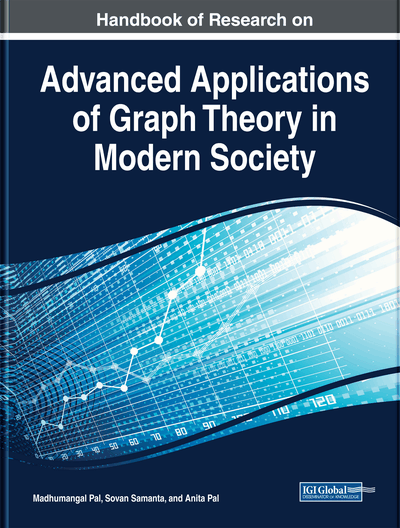# Set-Valuations of Graphs and Their Applications

Germina K. Augusthy (Central University of Kerala, India)
DOI: 10.4018/978-1-5225-9380-5.ch008
Available
\$37.50
No Current Special Offers

## Abstract

A set-valuation of a graph G=(V,E) assigns to the vertices or edges of G elements of the power set of a given nonempty set X subject to certain conditions. A set-indexer of G is an injective set-valuation f:V(G)→2x such that the induced set-valuation f⊕:E(G)→2X on the edges of G defined by f⊕(uv)=f(u)⊕f(v) ∀uv∈E(G) is also injective, where ⊕ denotes the symmetric difference of the subsets of X. Set-valued graphs such as set-graceful graphs, topological set-graceful graphs, set-sequential graphs, set-magic graphs are discussed. Set-valuations with a metric, associated with each pair of vertices is defined as distance pattern distinguishing (DPD) set of a graph (open-distance pattern distinguishing set of a graph (ODPU)) is ∅≠M⊆V(G) and for each u∈V(G), fM(u)={d(u,v): v ϵ M} be the distance-pattern of u with respect to the marker set M. If fM is injective (uniform) then the set M is a DPD (ODPU) set of G and G is a DPD (ODPU)-graph. This chapter briefly reports the existing results, new challenges, open problems, and conjectures that are abound in this topic.
Chapter Preview
Top

## Introduction

Labeling is a term used in technical sense for naming objects using symbolic format drawn from any universe of discourse such as the set of numbers, algebraic groups or the power setof a ‘ground set’ X. The objects requiring labeling could come from a variety of fields of human interest such as chemical elements, radio antennae, spectral bands and plant/animal species. Further, categorization of objects based on certain clustering rules might lead to derived labels from the labels of objects in each cluster; for instance labels a and b of two individual elements in a dyad {A,B} could be used to derive a labeling for the dyad in a way that could reflect a relational combination of the labels a and b. To be specific, A and B are assigned labels a,b from an algebraic group, whence the dyad {A,B} is assigned the label a*b where * is the group operation. Such assignments are generally motivated by a need to optimize on the number of symbols used to label the entire discrete structure so that the structure could be effectively encoded for handling its computerized analysis. In general, graph labelings, where the basic elements (i.e., vertices and/or edges) of a graph are assigned elements of a given set or subsets of a nonempty ‘ground set’ subject to given conditions, have often been motivated by practical considerations such as the one mentioned above. They are also of theoretical interest on their own right. ‘Graph labeling’ as an independent notion using numbers was first introduced in the mid sixties. Most graph labeling methods trace their origin to one introduced by Rosa (1967).

Even though the study of graceful graphs and graceful labeling methods was introduced by Rosa (1965) the term graceful graph was used first by Golomb (1972). Rosa (1965) defined a β-valuation of a (p,q)-graph G as an injection f from the vertices of G to the set {0,1,…,q−1} such that, when each edge xy is assigned the label |f(x)−f(y)|, the resulting edge labels are all distinct. In a graceful labeling of a graph G the resulting edge labels must be distinct and take values 1,2,…,q. The study of graceful labelings of a graph is a prolific area of research in graph theory. The graceful labeling problem is to determine which graphs are graceful. Proving a graph G is or is not graceful involves either producing a graceful labeling of G or showing that G does not admit a graceful labeling. While the graceful labeling of graphs is perceived to be a primarily theoretical topic in the field of graph theory, gracefully labelled graphs often serve as models in a wide range of applications. Such applications include coding theory and communication network addressing. Bloom and Golomb (1977) give a detailed account of some of the important applications of gracefully labelled graphs. That ‘all trees are graceful’ is a long-standing conjecture known as the “Ringel–Kotzig Conjecture” (Acharya, Rao & Arumugam, 2008).

## Complete Chapter List

Search this Book:
Reset